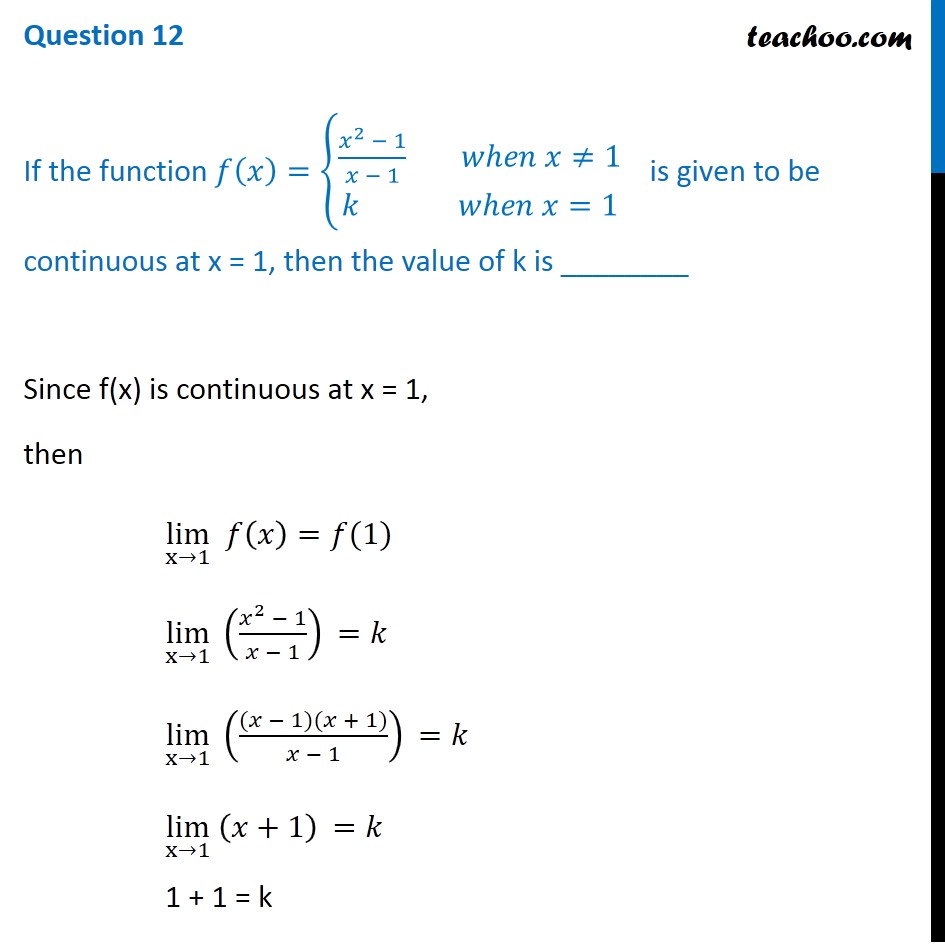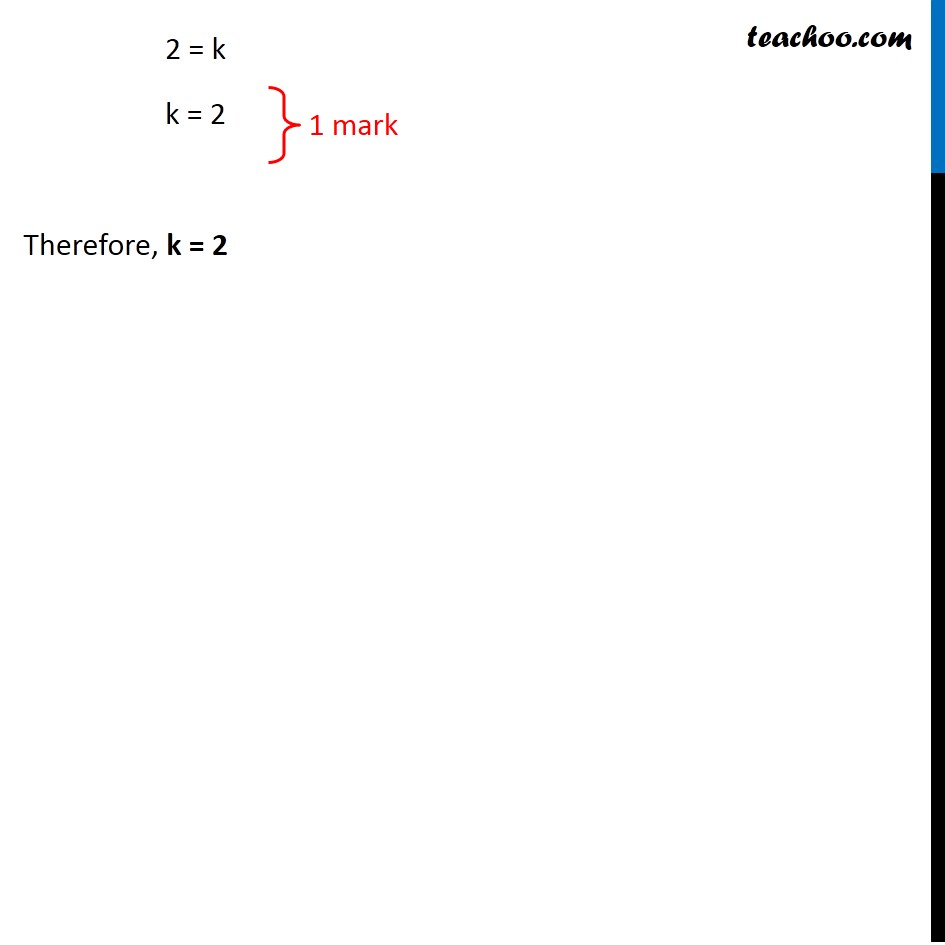If the function f (x) = {(x 2   - 1)/(x - 1) when x ≠ 1,  k when x = 1 } is given to be continuous at x = 1, then the value of k is ________1. Class 12
2. Solutions of Sample Papers and Past Year Papers - for Class 12 Boards
3. CBSE Class 12 Sample Paper for 2020 Boards

Transcript

Question 12 If the function 𝑓(𝑥)={(𝑥^2 − 1)/(𝑥 − 1) 𝑤ℎ𝑒𝑛 𝑥≠1 @𝑘 𝑤ℎ𝑒𝑛 𝑥=1 )┤is given to be continuous at x = 1, then the value of k is ________ Since f(x) is continuous at x = 1, then lim┬(x→1) 𝑓(𝑥)=𝑓(1) lim┬(x→1) ((𝑥^2 − 1)/(𝑥 − 1)) =𝑘 lim┬(x→1) (((𝑥 − 1)(𝑥 + 1))/(𝑥 − 1)) =𝑘 lim┬(x→1) (𝑥+1) =𝑘 1 + 1 = k 2 = k k = 2 Therefore, k = 2

CBSE Class 12 Sample Paper for 2020 Boards

Class 12
Solutions of Sample Papers and Past Year Papers - for Class 12 Boards